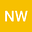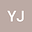Green H-relation of the square matrices over a local ring whose maximal ideal is generated by a nilpotent element
••• Nan Wangyu,
• Yang Jiang
Nan Wangyu
Shenyang Normal University
Author ProfileYang Jiang
Shenyang Normal University
Author Profile## Abstract

Let $R$ be a commutative local ring whose maximal ideal is generated by a nilpotent element, and $\Mat (n, R)$ be the multiplicative monoid of the square matrices of order $n$ over $R$. In this article, we provide (1) the construction of the Green’s $H$-equivalence classes in $\Mat (n, R)$, and (2) the enumeration of the Green’s $H$-equivalence classes in $\Mat (n, Z/ p^d Z)$.